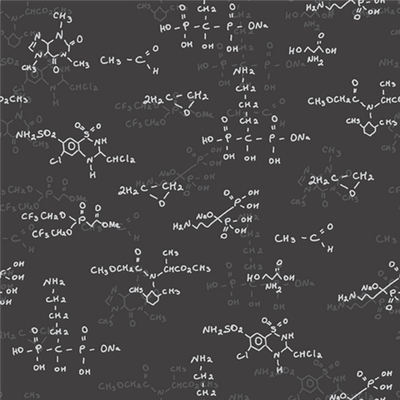### StoichiometryStoichiometry is a large word that simply means the mathematical side of chemistry. When a chemist has enough information, stoichiometry allows him to calculate masses, formulas and percents inside chemical equations.

Understanding Chemical Equations

Chemical equations are the foundation of stoichiometry. These involve the symbolic equation showing a chemical reaction. An example would be the following:

8Al + 3Fe3O4 → 4Al2O3 + 9Fe

Both sides of the chemical equation must have the same number of atoms of each element. In the above equation, each side has eight aluminum atoms, nine iron atoms, and twelve oxygen atoms. This equation is considered balanced. The large numbers in front of the elements are called coefficients, and one part of stoichiometry involves balancing equations with the addition of the right coefficients.

Percent Composition

Chemists can also use stoichiometry to determine percent of a compound. Percent composition is the percent of each element by mass that makes up the compound. This is found based on dividing the atomic mass of each element by the molecular mass (the total of the atomic mass of all atoms in the molecule). Water, which has two hydrogen atoms (atomic mass 1g each) and one oxygen atom (atomic mass 16) has a molecular mass of 18 g. to find the percent composition of the hydrogen, take its atomic mass (2 in this example) and divide it by the total molecular mass (18). This yields 11.1%. Repeat the process for oxygen to get 88.9%.

Empirical Formula

Empirical formula is the most "reduced" combination of a compound's chemical formula. For instance, H4O2 is a possible way to write the chemical composition of water, but the empirical formula is actually H2O, which is a simpler formula.

Molecular Formula

The molecular formula of a molecule is the form that the molecule usually uses in a chemical equation. While C3H9 is not as simple as possible, and therefore it is not an empirical formula, this is the way that it normally shows up in a chemical equation. Empirical formulas can be used to derive molecular formulas when the mass of an element in an equation is known.

### Subscribe Now!

Subscribe to our Free Experiment of the Month Club

*
*
*